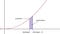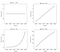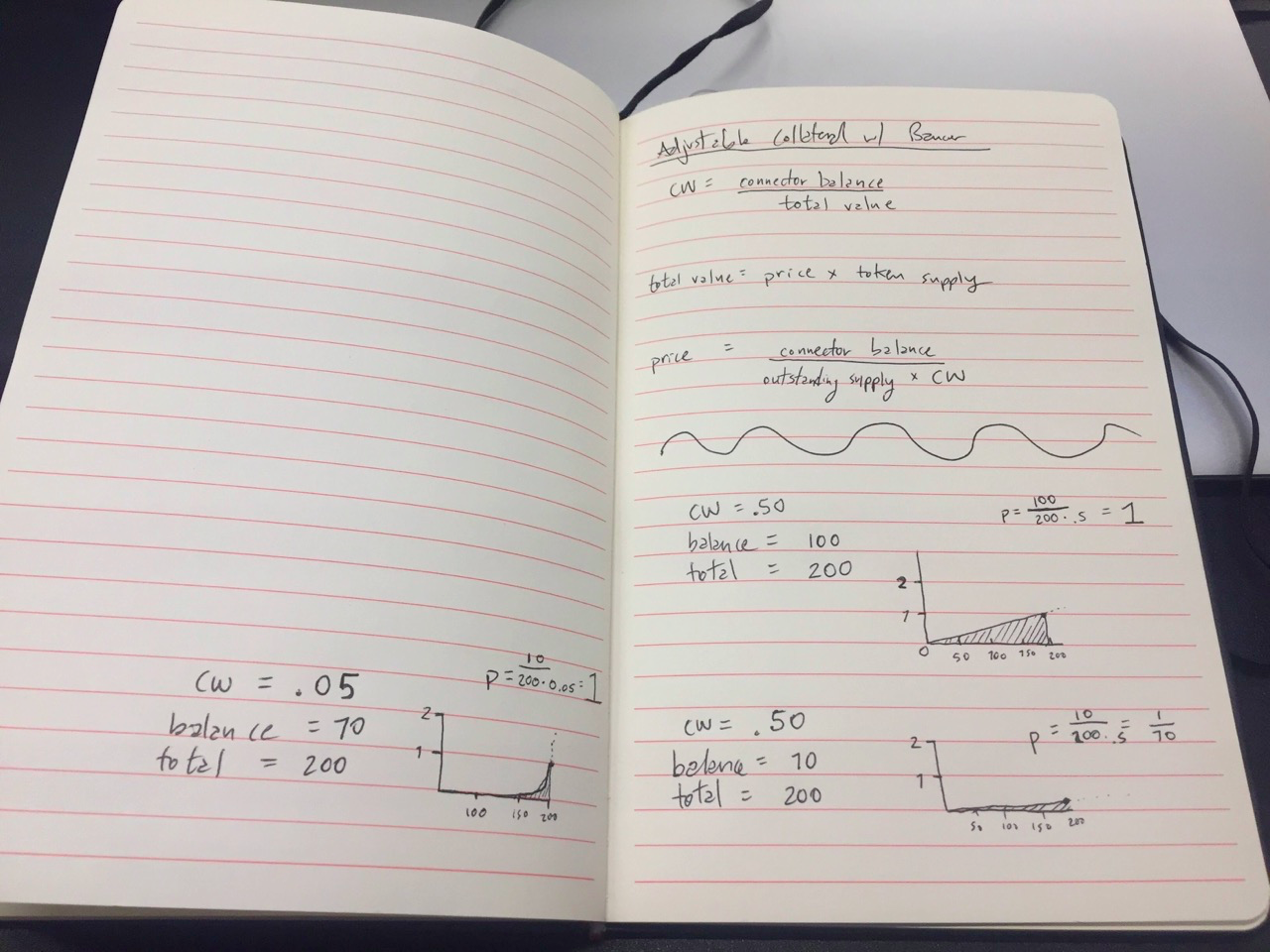# Converting Between Bancor and Bonding Curve Price FormulasPhoto by Wim van 't Einde on Unsplash

When dealing with automated market makers you come across a few different variations and use cases. You’ve got the Logarithmic Scoring Rule (LMSR) created by Robin Hanson and used in prediction markets like Gnosis. You’ve got xy=k proposed by Alan Lu and Vitalik Buterin on Reddit and built into Uniswap by Hayden Adams. Then you’ve got Simon de la Rouviere’s Bonding Curve Token and Bancor’s Smart Token. These last two are the ones I’d like to discuss today as they’re the most helpful when discussing Curation Markets. In this article I’ll go over the equations used in both paradigms and techniques for converting between them.

Curation markets “serve to reduce information asymmetry in the market through the usage of novel, skin-in-the-game signals generated through the use of tokenized cryptoeconomic incentive games” (source). For more information on the topic in general check out this, this or this. For the history of the concept and terminology check out Bancor’s Smart Tokens vs Token Bonding Curves.

# Connector Weight vs Slope Graph

Most of the time when discussing Curation Markets the term Bonding Curve or Bonded Curve is used to describe this special kind of automated market maker that burns and mints tokens according to some pre-defined price formula. While there are some proposals for more multi-faceted formulas, the discussion is mostly around ones with linear, quadratic and logarithmic curves.

This is helpful because it’s easy to describe these curves using simple slope graph formulas like `f(x) = mx`, `f(x) = mx^2` and `f(x) = mx^(1/2)` where `f(x)` is the price and `x` is the number of tokens in circulation. To calculate purchase and sale returns on these kinds of slope graphs you take the integral of the price formula then use it to calculate the area under the curve between two token amounts. The area under the curve represents the amount of collateral needed to mint or burn some amount of tokens.https://blog.relevant.community/how-to-make-bonding-curves-for-continuous-token-models-3784653f8b17

When dealing with a hostile environment like the Ethereum Virtual Machine (EVM) you’re forced to work without floating point numbers. This makes it difficult to design integral functions although some nice implementations do exist (link, link). Bancor found another method of calculating purchase and sale returns which unlocks some interesting and under-utilized features as a result.

Instead of taking the integral of a price formula, Bancor defined a constant called the Connector Weight (CW) which represents the ratio between the collateral and the market cap. Market cap is the current price per token multiplied by the total token supply. With these relationships in place you can extract a price formula consisting of the collateral, the token supply and the CW instead of using a fixed slope. You can read the proof that was generated in creating this method for price calculation here. In total you’re left with the the following equations:

• `CW = collateral / marketCap`
• `marketCap = price * tokenSupply`
• `price = collateral / (tokenSupply * CW)`

The following functions can be used to calculate the returns when buying and selling tokens:

• `buyAmt = tokenSupply * ((1 + amtPaid / collateral)^CW — 1)`
• `sellAmt = collateral * ((1 + tokensSold / totalSupply)^(1/CW) — 1)`

The interesting part of this method is that while the CW defines a family of curves, it does not define the exact slope of that curve. Instead, the values for `tokenSupply` and `collateral` ultimately effect the slope. This makes it possible to have a dynamic price curve that adjusts to inflation and deflation of a token. A token defined by a fixed slope only has exactly enough collateral to cover the exact number of tokens in circulation.

In order to utilize these dynamic price curves enabled by Connector Weight formulas it’s helpful to make the curves legible as slope graphs. The pieces necessary for this are `m` the slope and `n` the exponent so that you can make a formula like `f(x) = mx^n`. Both can be derived with the following equations:

• `CW = 1 / (n + 1)` where `n` is the exponent
• `m = collateral / (CW * tokenSupply ^ (1 / CW))`

In the example CWs from the Bancor whitepaper you would yield the following exponents as seen in the graphs below.

`a) CW = 1, n = 0 ------> f(x) = mb) CW = 1/2, n = 1 ----> f(x) = mxc) CW = 1/10, n = 9 ---> f(x) = mx^9d) CW = 9/10, n = 1/9 -> f(x) = mx^(1/9)`Bancor whitepaper

Here you can see that CW is the only thing that effects the exponent of the price formula. When you look at the `m` slope of the price formula again, `m = collateral / (CW * tokenSupply ^ (1 / CW))`, you can see that it is the CW, the token supply as well as the collateral which have an effect. This gives you the freedom to adjust your price formula dynamically by altering any of those values.

For example, you may want to use some of the locked collateral in a bonding curve for operation costs. In this scenario you could allow it to be removed without burning any tokens from the supply. This would lower the collateral but keep the token supply the same. In the same vein you might keep the collateral the same but increase the token supply as a form of inflation or some kind of reward. Either way you’re confronted with a decision regarding the CW where you have two options:

1. You could keep the CW the same but as a result watch the price per token drop. You would decrease the slope of the price graph, but you would preserve the exponent that was used in the calculation. This would basically preserve the `n` while altering the `m` of a formula like `f(x) = mx^n`. Since the exponent will have a larger effect on the price than the slope it will preserve qualities of your token’s volatility and price slippage, but it will lower the immediate price per token.
2. You could also change the CW after removing collateral so that the price per token stays the same. In doing this you would change the exponent that is used for calculating the price as well as the slope, pushing linear curves towards exponential curves and making exponential curves crazy exponential. This would exacerbate the qualities of your token’s volatility and increase price slippage, but it will preserve the immediate price per token.

Both scenarios are expressed with chicken scratches in the notebook below. The graph at the top of the three represents an initial state of a bonding curve. Below are the scenarios where collateral has left the contract. On the left the CW was decreased in order to keep the current price the same. You can see that the graph went from linear to exponential. On the right you can see that the CW was kept the same and as a result the current price per token dropped, although the linear quality of the graph was preserved. The scenario could similarly be reversed where collateral is added without minting tokens, or tokens are burned without redeeming collateral. This would have the opposite effect of decreasing the volatility and price slippage, potentially creating a more stable ecosystem for your token holders.The two bottom graphs represent the same loss of collateral, the one on the left alters the CW to preserve the current price and the one on the right preserves the CW instead. You can see that the area under the graphs representing the collateral in the contract are equal in both of the bottom scenarios.

# Takeaway

Hopefully this quick overview is helpful while thinking about Bonding Curves and the different methods available for implementing them. The implications of these methods have far reaching applications. One of the most exciting ones to me is with regard to DAOs, organizations and communities which could use these kinds of curves for continuous funding opportunities and/or crowd sourced equity. Take a look at projects like Apiary and Continuous Organizations for more info.

For an amazing deep dive into Bonding Curve math and mechanics take a look at the article Slava Balasanov published this week called Bonding Curves In Depth: Intuition & Parametrization.

My name is Billy Rennekamp. I’m a co-founder of Bin Studio, a multidisciplinary research, design and development studio based in Berlin. We’re currently developing Clovers Network, an NFT art game supported by the ECF — Ethereum Community Fund. I work on a lot of other great projects including Cosmos Network, Gnosis, ENS Nifty, Meme Lordz and Doneth. If any of that sounds interesting to you, follow my twitter, my github or just say hello 👋

https://okw.me